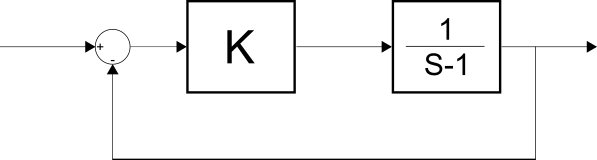# A Stable plan

For what value of $n$ will the system presented in the image be stable for a $k > n?$ Consider $n$ to be the smallest possible value of $n.$×